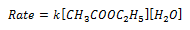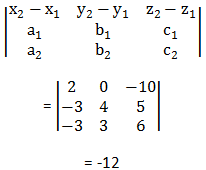# Coplanarity of Two Lines In 3D Geometry

Two lines are said to be coplanar when they both lie on the same plane in a three-dimensional space. We have learnt how to represent the equation of a line in three-dimensional space using vector notations. In this article, we will learn about coplanarity of two lines.

#### Condition for coplanarity of two lines in vector form:

Using vector notations equation of line is given by:

$\vec{r}$ = $\vec{l_{1}}$ + λ$\vec{m_{1}}$       ——————— (1)

$\vec{r}$ = $\vec{l_{2}}$ + μ$\vec{m_{2}}$  ——————– (2)

Here, the line (1) passes through a point L having position vector $\vec{l_{1}}$ and is parallel to $\vec{m_{1}}$ and the line (2) passes through a point M having position vector $\vec{l_{2}}$ and is parallel to $\vec{m_{2}}$. These two lines are coplanar if and only if $\vec{LM}$ is perpendicular to $\vec{m_{1}}$ x $\vec{m_{2}}$.

This can be given as:

$\vec{LM}$ = $\vec{l_{2}}$$\vec{l_{1}}$

Thus condition of coplanarity is given by:

$\vec{LM}$.($\vec{m_{1}}$ x $\vec{m_{2}}$) = 0
($\vec{l_{2}}$$\vec{l_{1}}$).($\vec{m_{1}}$ x $\vec{m_{2}}$) = 0

#### Condition for coplanarity of two lines in cartesian form:

Let us take two points L and M such that (x1, y1, z1) and (x2, y2, z2) be the coordinates of the points respectively. The direction cosines of two vectors $\vec{m_{1}}$ and $\vec{m_{2}}$ is given by a1, b1, c1 and a2, b2, c2 respectively.

$\vec{LM}$ = (x2 − x1)$\hat{i}$ + (y2 − y1)$\hat{j}$ + (z2 − z1)$\hat{k}$

$\vec{m_{1}}$ = a1$\hat{i}$  +b1$\hat{j}$   + c1$\hat{k}$

$\vec{m_{2}}$ = a2$\hat{i}$  +b2$\hat{j}$  + c2$\hat{k}$

By the above condition two lines can be coplanar if and only if,

$\vec{LM}$.($\vec{m_{1}}$ x $\vec{m_{2}}$) = 0

This can be represented in cartesian form as,#### Problems related to coplanarity of two lines:

Question: Show that lines $\frac{x + 3}{-3}$ = $\frac{y – 2}{4}$ = $\frac{z – 5}{5}$ and $\frac{x + 1}{-3}$ = $\frac{y – 2}{3}$ = $\frac{z + 5}{6}$ are coplanar.

Solution:

According to the question:

x1 = -3, y1 = 2, z1 = 5, x2 = -1 , y2 = 2, z2 = -5, a1 = -3 , b1 = 4, c1 = 5, a2 = -3 , b2 = 3, c2 = 6